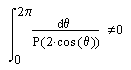Am Math Monthly 71 (1964), 180

## Yet Another Proof of the FundamentalTheorem of Algebra

R. P. Boas, Jr.

The following proof of the fundamental theorem of algebra by contour integration is similar to Ankeny's , but is simpler because it uses integration around the unit circle (which is usually the first application of contour integration) instead of integration along the real axis; thus there is no need to discuss the asymptotic behavior of any integrals.

Let P(z) be a nonconstant polynomial; we are to show that P(z) = 0 for some z. We may suppose P(z) real for real z. (Indeed, otherwise letbe the polynomial whose coefficients are the conjugates of those of P(z) and consider.) Suppose then that P(z) is real for real z and is never 0; we deduce a contradiction. Since P(z) does not either vanish or change sign for real z, we have

 (1)But this integral is equal to the contour integral
 (2)where Q(z) = znP(z + z-1) is a polynomial. For z ≠ 0, Q(z)≠0; in addition, if an is the leading coefficient in P(z), we have Q(0) = an ≠0. Since Q(z) is never zero, the integrand in (2) is analytic and hence the integral is zero by Cauchy's theorem, contradicting (1).

### Reference

1. N.C. Ankeny, One more proof of the fundamental theorem of algebra, this Monthly, 54 (1947) 464.